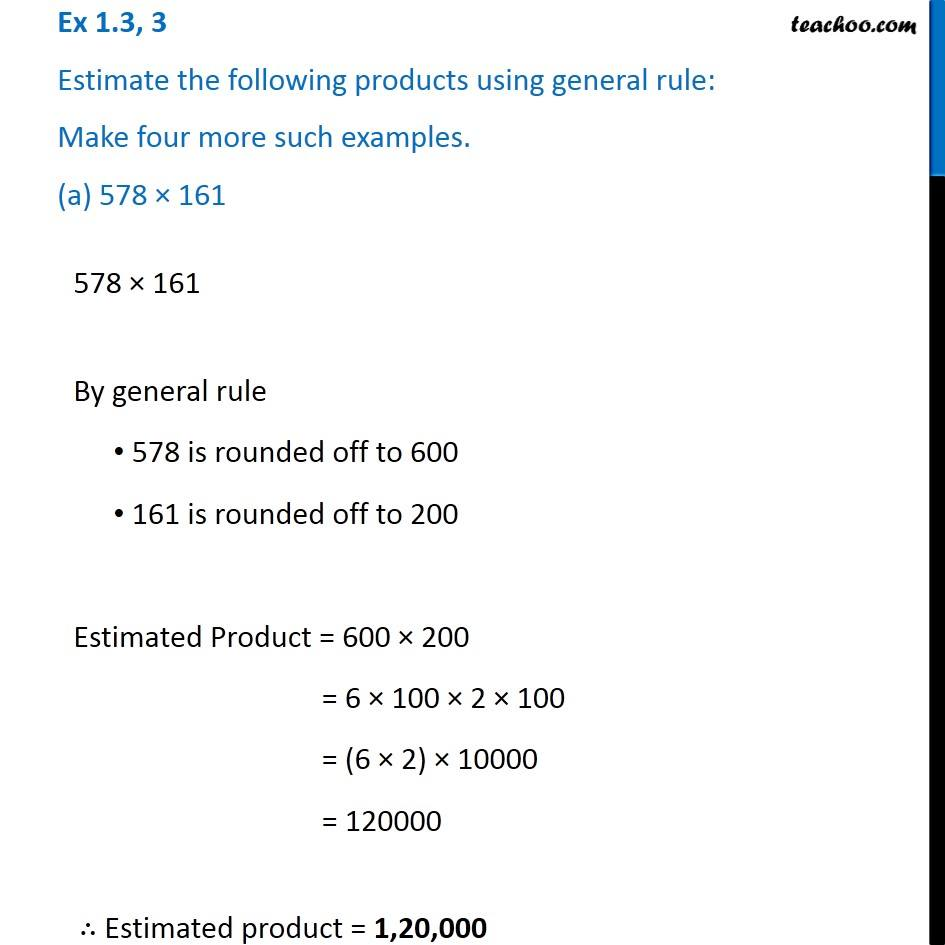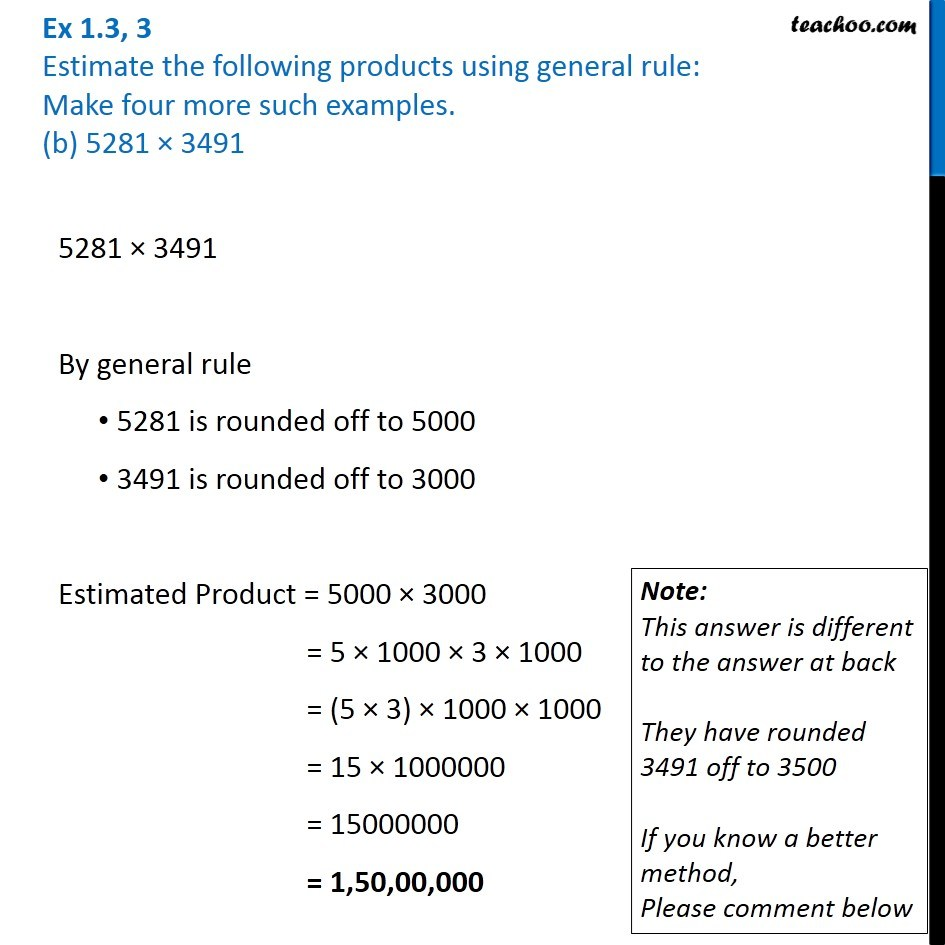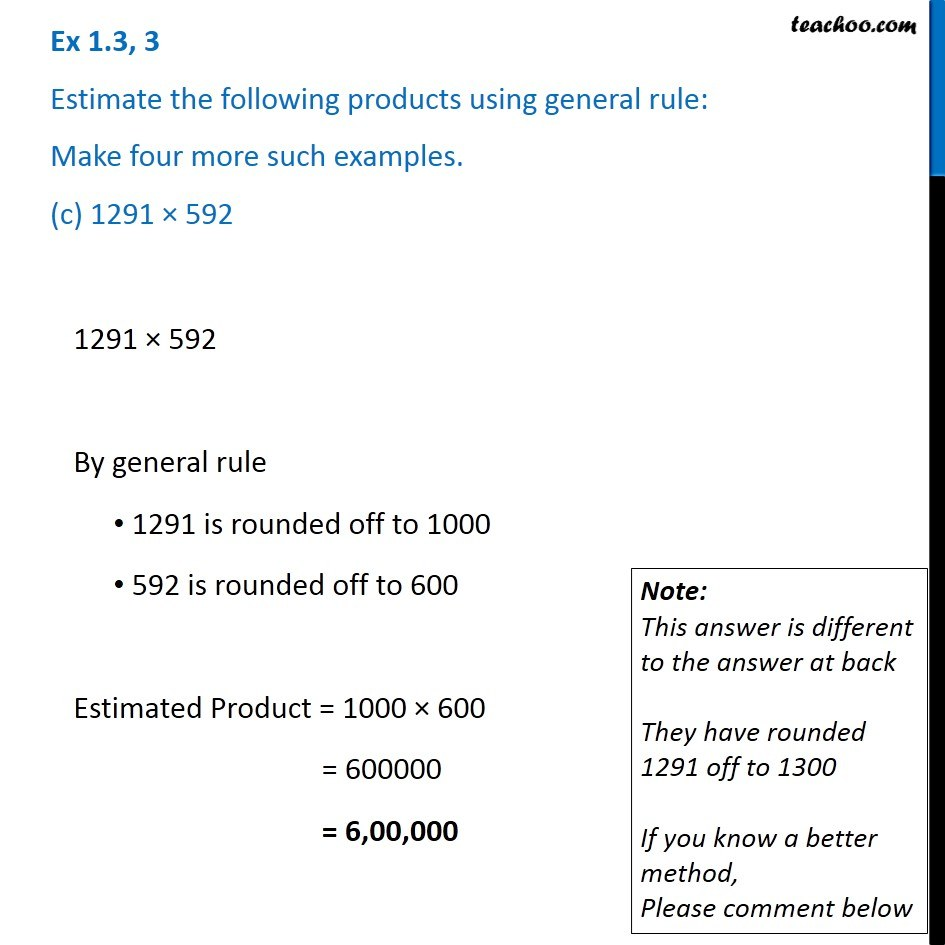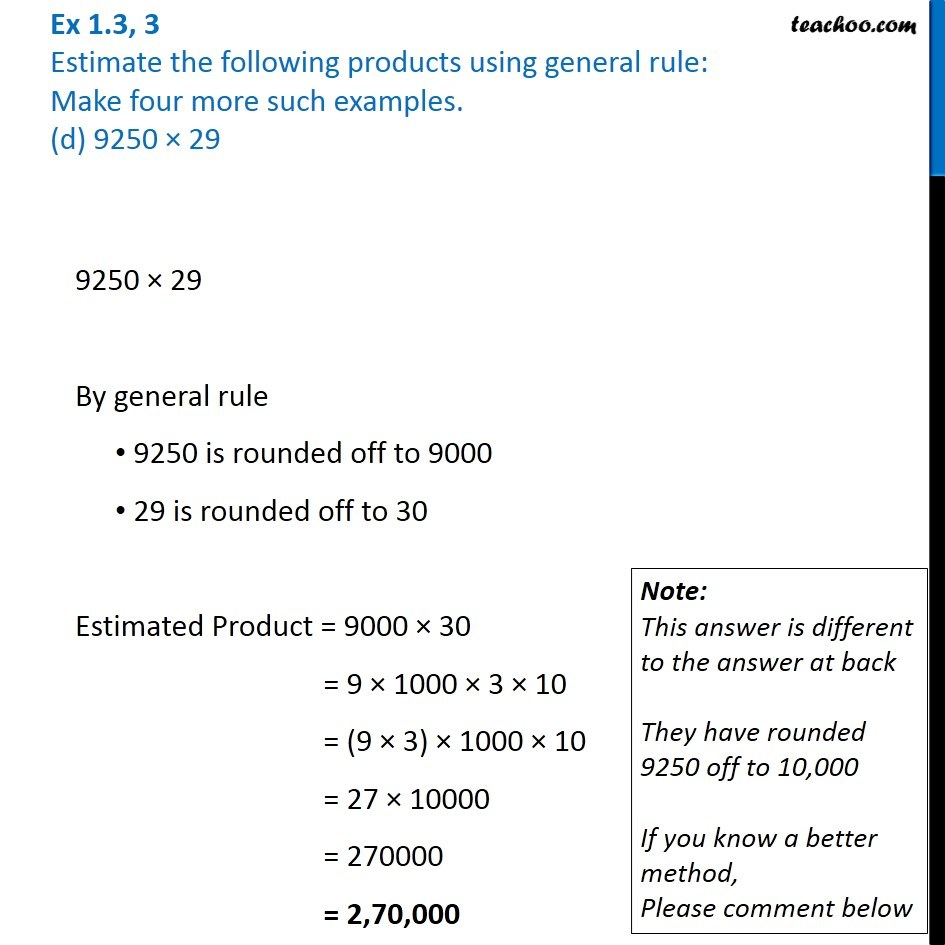Subscribe to our Youtube Channel - https://you.tube/teachoo

1. Chapter 1 Class 6 Knowing our Numbers
2. Concept wise

Transcript

Ex 1.3, 3 Estimate the following products using general rule: Make four more such examples. (a) 578 × 161578 × 61 By general rule 578 is rounded off to 600 161 is rounded off to 200 Estimated Product = 600 × 200 = 6 × 100 × 2 × 100 = (6 × 2) × 10000 = 120000 ∴ Estimated product = 1,20,000 Ex 1.3, 3 Estimate the following products using general rule: Make four more such examples. (b) 5281 × 34915281 × 3491 By general rule 5281 is rounded off to 5000 3491 is rounded off to 3000 Estimated Product = 5000 × 3000 = 5 × 1000 × 3 × 1000 = (5 × 3) × 1000 × 1000 = 15 × 1000000 = 15000000 = 1,50,00,000 Note: This answer is different to the answer at back They have rounded 3491 off to 3500 If you know a better method, Please comment below Ex 1.3, 3 Estimate the following products using general rule: Make four more such examples. (c) 1291 × 5921291 × 592 By general rule 1291 is rounded off to 1300 592 is rounded off to 600 Estimated Product = 1300 × 600 = 13 × 6 × 10000 = 780000 = 7,80,000 Ex 1.3, 3 Estimate the following products using general rule: Make four more such examples. (d) 9250 × 299250 × 29 By general rule 9250 is rounded off to 9000 29 is rounded off to 30 Estimated Product = 9000 × 30 = 9 × 1000 × 3 × 10 = (9 × 3) × 1000 × 10 = 27 × 10000 = 270000 = 2,70,000 Note: This answer is different to the answer at back They have rounded 9250 off to 10,000 If you know a better method, Please comment below